# Python基础-字符串(字符串常用函数/操作/字符串遍历)### 常用函数/操作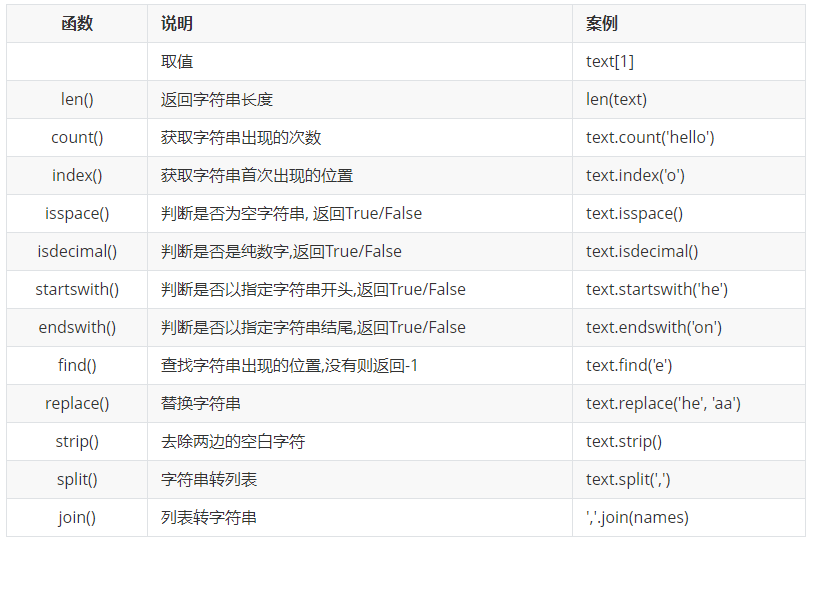``````text = 'abcde'
print(text)``````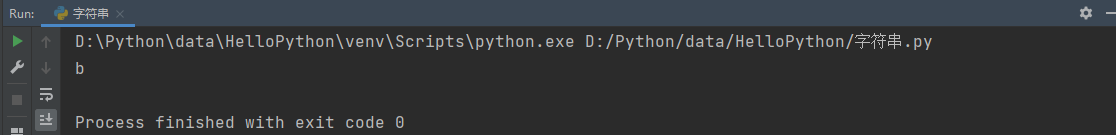len(), 获取字符串的长度

``````text = 'hello'
print('字符串的长度是:% d' % len(text))``````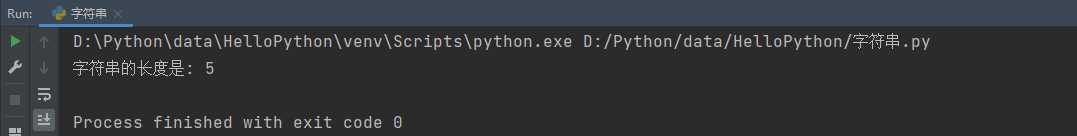count(), 获取指定字符或字符串在字符串中出现的次数

``````text = 'abc abc'
print('字符串ab在字符串中出现的次数是:%d' % text.count('ab'))``````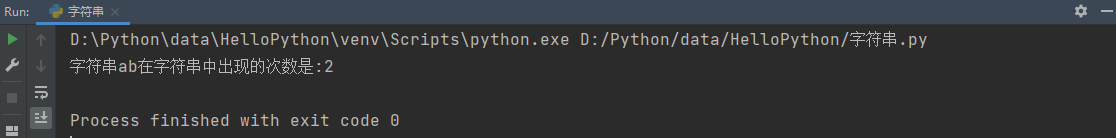index(), 获取指定字符或字符串在字符串中首次出现的位置

``````text = 'abc abc'
print('字符串ab在字符串中首次出现的位置是:%d' % text.index('ab'))``````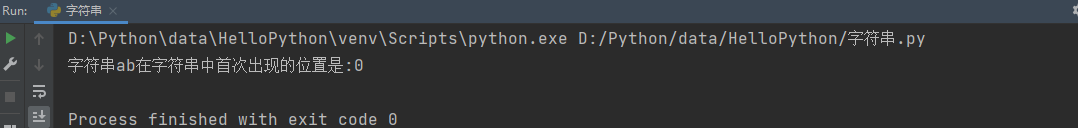### 判断类型

isspace(), 判断字符串是否是空白字符串( 是则返回True, 不是则返回False)

``````text = ' '
print('一个空格是否为空白字符:%s' % text.isspace())
text = '      '
print('多个空格是否为空白字符:%s' % text.isspace())
text = '   a'
print('包含空格和字母是否为空白字符:%s' % text.isspace())``````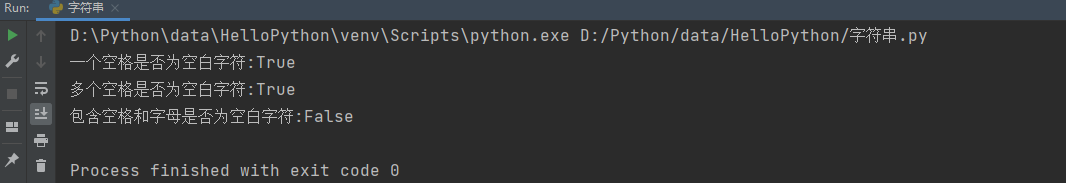isdecimal(), 判断字符串是否是纯数字( 是则返回True, 不是则返回False)

``````text = '123'
print('字符串123 是否是纯数字:%s' % text.isdecimal())
text = '123a'
print('字符串123a 是否是纯数字:%s' % text.isdecimal())``````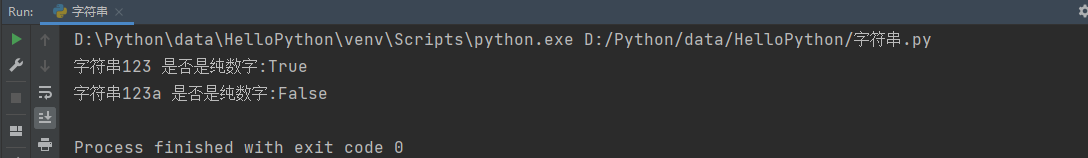startwith(), 判断字符串是否以指定字符串开头( 是则返回True, 不是则返回False)

``````text = 'hello python'
print('字符串hello python 是否以he开头: %s' % text.startswith('he'))``````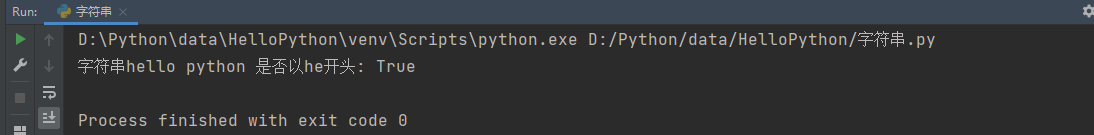endswith(), 判断字符串是否已指定字符串结尾( 是则返回True, 不是则返回False)

``````text = 'hello python'
print('字符串hello python 是否以on结尾: %s' % text.endswith('on'))``````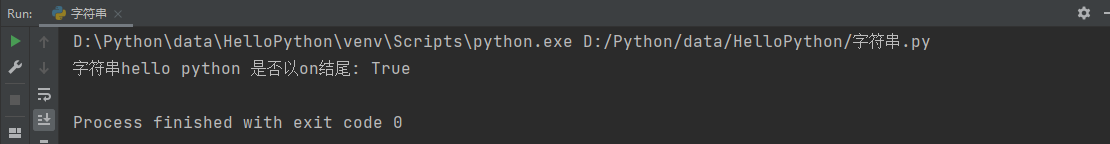find(), 查找指定字符串在字符串中出现的位置( 有则返回字符串所在的索引位置, 没有则返回-1)

``````text = 'hello python'
print('在字符串hello python 查找字符串e 出现的位置: %s' % text.find('e'))
print('在字符串hello python 查找字符串a 出现的位置: %s' % text.find('a'))``````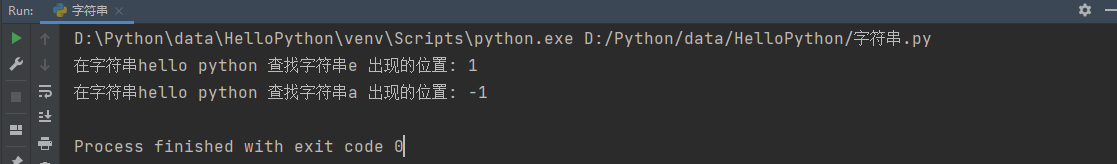replace(), 替换字符串( 第一个参数写需要被替换的字符串, 第二个参数写替换后的字符串)

``````text = 'hello python'
print(text.replace('he', 'aa'))``````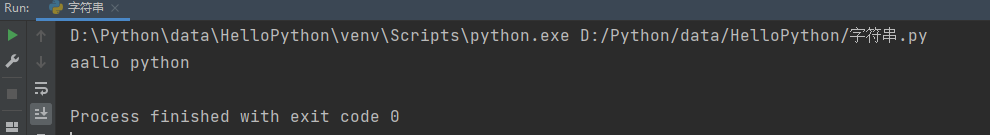strip(), 去除字符串来两边的空白字符

``````text = '   hello python   '
print('去除空格前:%s' % text)
print('去除空格后:%s' % text.strip())``````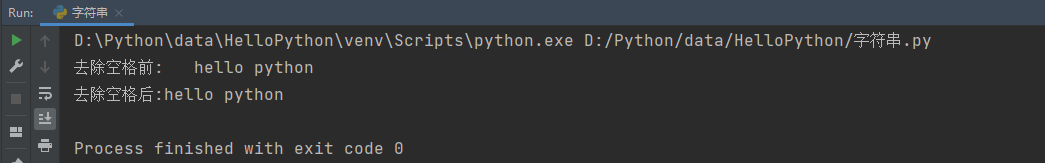split(), 将字符串按照指定分隔符, 转换成列表

``````text = '张三,李四,王五,赵六'
names = text.split(',')
print(names)``````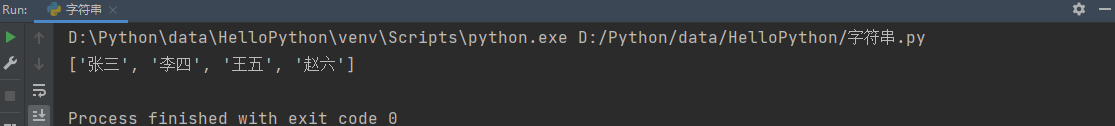join(), 按照指定分隔符, 将列表转换成字符串

``````names = ['张三', '李四', '王五', '赵六']
print(','.join(names))``````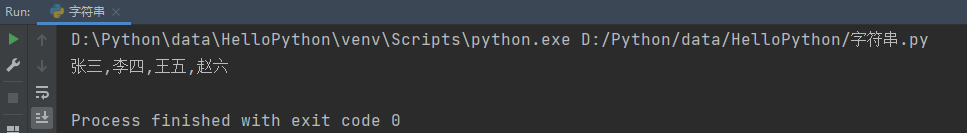-- 展开阅读全文 --

Redis底层数据结构--跳跃表
« 上一篇 04-28
BUUCTF Web [强网杯 2019]随便注